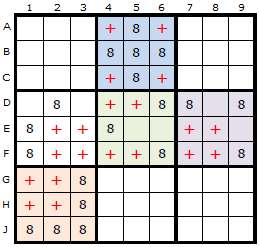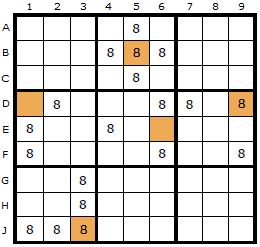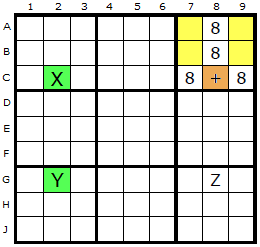Main Page - Back

# Empty Rectangles

From sudokuwiki.org, the puzzle solver's siteEmpty Rectangles can be re-expressed as Grouped X-Cycles. Examples are given below. In order to see the Empty Rectangle the tick-box on the solver for Grouped X-Cycles must be off. Counter-examples would be appreciated if discovered.Empty Rectangles in Boxes Empty Rectangles constitute a clever but obscure strategy for Sudoku. An Empty Rectangle occurs within a box where four cells form a rectangle which does NOT contain a certain candidate.

In the first diagram is a spread of candidate 8 around the board but each box must be considered separately when imagining the rectangle, so I have coloured the boxes differently. To be able to apply this strategy we must have at least two candidates left in the box and four or more empty cells.

The red crosses represent the Empty Rectangles. Each of these theoretical rectangles is independent of the others.
Whatever the formation of the Empty Rectangle (ER), we will have a vertical and horizontal gap where we can imagine two Empty Rectangle Lines (ERLs). These are two lines we can draw inside the box without touching the ER itself.

I have picked box two in this example.Empty Rectangle Intersections We can quickly forget about these lines once we've spotted where they cross - a place known as the Empty Rectangle Intersection (ERI).

In this diagram the ERIs have been marked with a brown cell.Example ERI arrangement If you have a strong link and an ERI that shares a line (row or column), then you can eliminate a candidate at that point where the other end of the strong link and the ERI intersect. Let's look at some visual examples of the formation. Two different arrangements are shown in this and the next diagram.

The plus sign now indicates where the Empty Rectangle Intersection (ERI) is placed. In the first example down column 2, two 8s exist and form a strong link (X and Y). One end of the strong link (X at C2) can see the ERI at C8.
In the second example the ERI at A7 can see an 8 in A4 (the X) and this is paired with the 8 on the cell marked Y. Y can see the 8 in Z which in turn is visible to the original ERI.

It is proposed in both examples that the 8 in Z can be removed.Empty Rectangle Example : Load Example or : From the Start Here is a real example based on the centre box. The remaining options for 6 in box 5 is D4, D5, E6 and F6. This gives us an Empty Rectangle shown by the four yellow cells. The Empty Rectangle Intersection is the orange cell on D6. Our X and Y are the two green cells H6 and H9. It doesn't matter that there are a ton of 6s in column 6, what is important is there are two 6s in row H. H6 can see the ERI and H9 can see the intersection of H9 and the ERI - which is D9. That is the 6 we can remove.